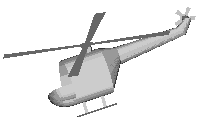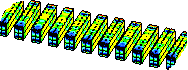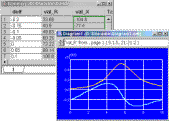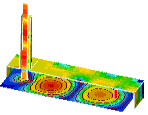Electro
Dynamics of
Elements of
Metall

# a program for electromagnetic fields calculating and researching into the electrodynamic properties of conducting structures

 EDEM (ElectroDynamics of Elements of Metal with 3-Dimensional geometry) is designed for specialists in the field of Applied Electromagnetics. The software allows the possibility of electromagnetic fields calculations and investigating the electrodynamical properties of structures which may be represented by set of perfectly conducting surfaces. Rigorous integral equations and method of moments are used for solving the problems. The analyzed objects can be of practically arbitrary shape. For their approximation polygons, spirals, disks, rings and their sectors, closed and unclosed surfaces of revolution and cylindrical surfaces, formed by curves of the second order and two-dimentional splines, and also the surfaces formed by three-dimensional splines are used. Objects can contain absorbing and impedance films, lumped capacities and inductances, and also active loads.For solving tasks, their symmetry can effectively be used. It allows significant economy of memory and time of calculations. For example, when using three degrees of symmetry it is required only 1.5% of a memory size in comparison with the direct solving. Analyzed structures can form infinite periodic arrays. The integral equations at the solving of a problem use one period only of such arrays. The electromagnetic fields then can be computed by arbitrary number of periods.For the numerical solution it can be used both constant approximation, and the basic functions of higher order known as RWG functions. For the solving of systems of linear algebraic equations both direct and iterative methods are realized, in particular the Generalized Minimal Residual Algorithm (GMREZ). The analysis of problems for complex structures can be organized on the basis of a step-by-step method when results of the solving for a part of structure are used as incident field for other parts. The program allows to carry out the solving of tasks series in an automatic mode and display the results in a graphic and tabulare form. It releases from tiresome routine work at calculation of frequency characteristics, etc. The program includes instruments for optimization of researched structures by the given target criterion. It can be the directed gain of  antenna, etc. For this purpose polyvalent algorithms of functions extremum seeking are used.An approximate solving of tasks on the basis of a method of physical optics is possible. The sizes of researched structures can be at this very large.EDEM allows the possibility to determine the density of surface currents on these structures, near electromagnetic field prediction, far field pattern calculating, electromagnetic fields and Pointing's vector shapes constructing, level lines, magnitude and phase diagrams plotting, total radiating power and directive gain estimation, phase center and polarization characteristic determinations, and many other parameters. Using the rigorous equations guarantees that all the physical phenomena that is present in any system being analyzed is accounted for and high accuracy results are achieved.
 Contacts:  info@edem3d.ruSite design and support: Defi